# NCERT solutions for Mathematics Exemplar Class 8 chapter 5 - Understanding Quadrilaterals and Practical Geometry [Latest edition]

#### Chapters## Chapter 5: Understanding Quadrilaterals and Practical Geometry

Exercise
Exercise [Pages 144 - 163]

### NCERT solutions for Mathematics Exemplar Class 8 Chapter 5 Understanding Quadrilaterals and Practical Geometry Exercise [Pages 144 - 163]

#### Choose the correct alternative:

Exercise | Q 1 | Page 144

If three angles of a quadrilateral are each equal to 75°, the fourth angle is ______.

• 150°

• 135°

• 45°

• 75°

Exercise | Q 2 | Page 144

For which of the following, diagonals bisect each other?

• Square

• Kite

• Trapezium

Exercise | Q 3 | Page 144

For which of the following figures, all angles are equal?

• Rectangle

• Kite

• Trapezium

• Rhombus

Exercise | Q 4 | Page 144

For which of the following figures, diagonals are perpendicular to each other?

• Parallelogram

• Kite

• Trapezium

• Rectangle

Exercise | Q 5 | Page 144

For which of the following figures, diagonals are equal?

• Trapezium

• Rhombus

• Parallelogram

• Rectangle

Exercise | Q 6 | Page 144

Which of the following figures satisfy the following properties?
- All sides are congruent.
- All angles are right angles.
- Opposite sides are parallel.

•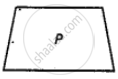•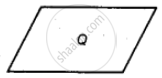••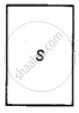Exercise | Q 7 | Page 145

Which of the following figures satisfy the following property?
- Has two pairs of congruent adjacent sides.

•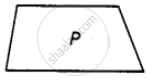•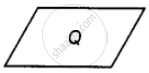•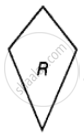•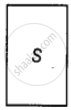Exercise | Q 8 | Page 145

Which of the following figures satisfy the following property?
- Only one pair of sides are parallel.

•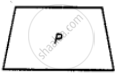••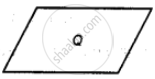•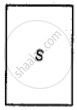Exercise | Q 9 | Page 145

Which of the following figures do not satisfy any of the following properties?
- All sides are equal.
- All angles are right angles.
- Opposite sides are parallel.

•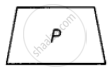•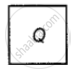•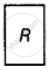•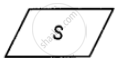Exercise | Q 10 | Page 145

Which of the following properties describe a trapezium?

• A pair of opposite sides is parallel

• The diagonals bisect each other

• The diagonals are perpendicular to each other

• The diagonals are equal

Exercise | Q 11 | Page 146

Which of the following is a property of a parallelogram?

• Opposite sides are parallel

• The diagonals bisect each other at right angles

• The diagonals are perpendicular to each other

• All angles are equal

Exercise | Q 12 | Page 146

What is the maximum number of obtuse angles that a quadrilateral can have?

• 1

• 2

• 3

• 4

Exercise | Q 13 | Page 146

How many non-overlapping triangles can we make in a n-gon (polygon having n sides), by joining the vertices?

• n – 1

• n – 2

• n – 3

• n – 4

Exercise | Q 14 | Page 146

What is the sum of all the angles of a pentagon?

• 180°

• 360°

• 540°

• 720°

Exercise | Q 15 | Page 146

What is the sum of all angles of a hexagon?

• 180°

• 360°

• 540°

• 720°

Exercise | Q 16 | Page 146

If two adjacent angles of a parallelogram are (5x – 5)° and (10x + 35)°, then the ratio of these angles is ______.

• 1 : 3

• 2 : 3

• 1 : 4

• 1 : 2

Exercise | Q 17 | Page 146

A quadrilateral whose all sides are equal, opposite angles are equal and the diagonals bisect each other at right angles is a ______.

• Rhombus

• Parallelogram

• Square

• Rectangle

Exercise | Q 18 | Page 146

A quadrilateral whose opposite sides and all the angles are equal is a ______.

• Rectangle

• Parallelogram

• Square

• Rhombus

Exercise | Q 19 | Page 146

A quadrilateral whose all sides, diagonals and angles are equal is a ______.

• Square

• Trapezium

• Rectangle

• Rhombus

Exercise | Q 20 | Page 147

How many diagonals does a hexagon have?

• 9

• 8

• 2

• 6

Exercise | Q 21 | Page 147

If the adjacent sides of a parallelogram are equal then parallelogram is a ______.

• Rectangle

• Trapezium

• Rhombus

• Square

Exercise | Q 22 | Page 147

If the diagonals of a quadrilateral are equal and bisect each other, then the quadrilateral is a ______.

• Rhombus

• Rectangle

• Square

• Parallelogram

Exercise | Q 23 | Page 147

The sum of all exterior angles of a triangle is ______.

• 180°

• 360°

• 540°

• 720°

Exercise | Q 24 | Page 147

Which of the following is an equiangular and equilateral polygon?

• Square

• Rectangle

• Rhombus

• Right triangle

Exercise | Q 25 | Page 147

Which one has all the properties of a kite and a parallelogram?

• Trapezium

• Rhombus

• Rectangle

• Parallelogram

Exercise | Q 26 | Page 147

The angles of a quadrilateral are in the ratio 1 : 2 : 3 : 4. The smallest angle is ______.

• 72°

• 144°

• 36°

• 18°

Exercise | Q 27 | Page 147

In the trapezium ABCD, the measure of ∠D is ______.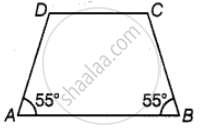• 55°

• 115°

• 135°

• 125°

Exercise | Q 28 | Page 147

A quadrilateral has three acute angles. If each measures 80°, then the measure of the fourth angle is ______.

• 150°

• 120°

• 105°

• 140°

Exercise | Q 29 | Page 147

The number of sides of a regular polygon where each exterior angle has a measure of 45° is ______.

• 8

• 10

• 4

• 6

Exercise | Q 30 | Page 148

In a parallelogram PQRS, if ∠P = 60°, then other three angles are ______.

• 45°, 135°, 120°

• 60°, 120°, 120°

• 60°, 135°, 135°

• 45°, 135°, 135°

Exercise | Q 31 | Page 148

If two adjacent angles of a parallelogram are in the ratio 2 : 3, then the measure of angles are ______.

• 72°, 108°

• 36°, 54°

• 80°, 120°

• 96°, 144°

Exercise | Q 32 | Page 148

If PQRS is a parallelogram, then ∠P – ∠R is equal to ______.

• 60°

• 90°

• 80°

Exercise | Q 33 | Page 148

The sum of adjacent angles of a parallelogram is ______.

• 180°

• 120°

• 360°

• 90°

Exercise | Q 34 | Page 148

The angle between the two altitudes of a parallelogram through the same vertex of an obtuse angle of the parallelogram is 30°. The measure of the obtuse angle is ______.

• 100°

• 150°

• 105°

• 120°

Exercise | Q 35 | Page 148

In the given figure, ABCD and BDCE are parallelograms with common base DC. If BC ⊥ BD, then ∠BEC = ______.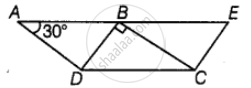• 60°

• 30°

• 150°

• 120°

Exercise | Q 36 | Page 148

Length of one of the diagonals of a rectangle whose sides are 10 cm and 24 cm is ______.

• 25 cm

• 20 cm

• 26 cm

• 3.5 cm

Exercise | Q 37 | Page 148

If the adjacent angles of a parallelogram are equal, then the parallelogram is a ______.

• Rectangle

• Trapezium

• Rhombus

• Any of the three

Exercise | Q 38 | Page 148

Which of the following can be four interior angles of a quadrilateral?

• 140°, 40°, 20°, 160°

• 270°, 150°, 30°, 20°

• 40°, 70°, 90°, 60°

• 110°, 40°, 30°, 180°

Exercise | Q 39 | Page 149

The sum of angles of a concave quadrilateral is ______.

• More than 360°

• Less than 360°

• Equal to 360°

• Twice of 360°

Exercise | Q 40 | Page 149

Which of the following can never be the measure of exterior angle of a regular polygon?

• 22°

• 36°

• 45°

• 30°

Exercise | Q 41 | Page 149

In the figure, BEST is a rhombus, Then the value of y – x is ______.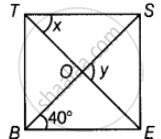• 40°

• 50°

• 20°

• 10°

Exercise | Q 42 | Page 149

The closed curve which is also a polygon is ______.

•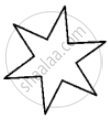•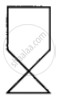•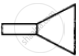•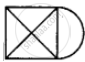Exercise | Q 43 | Page 149

Which of the following is not true for an exterior angle of a regular polygon with n sides?

• Each exterior angle = 360^circ/n

• Exterior angle = 180° – interior angle

• n = 360^circ/"exterior angle"

• Each exterior angle = ((n - 2) xx 180^circ)/n

Exercise | Q 44 | Page 149

PQRS is a square. PR and SQ intersect at O. Then ∠POQ is a ______.

• Right angle

• Straight angle

• Reflex angle

• Complete angle

Exercise | Q 45 | Page 150

Two adjacent angles of a parallelogram are in the ratio 1:5. Then all the angles of the parallelogram are ______.

• 30°, 150°, 30°, 150°

• 85°, 95°, 85°, 95°

• 45°, 135°, 45°, 135°

• 30°, 180°, 30°, 180°

Exercise | Q 46 | Page 150

A parallelogram PQRS is constructed with sides QR = 6 cm, PQ = 4 cm and ∠PQR = 90°. Then PQRS is a ______.

• Square

• Rectangle

• Rhombus

• Trapezium

Exercise | Q 47 | Page 150

The angles P, Q, R and S of a quadrilateral are in the ratio 1:3:7:9. Then PQRS is a ______.

• Parallelogram

• Trapezium with PQ || RS

• Trapezium with QR || PS

• kite

Exercise | Q 48 | Page 150

PQRS is a trapezium in which PQ || SR and ∠P = 130°, ∠Q = 110°. Then ∠R is equal to ______.

• 70°

• 50°

• 65°

• 55°

Exercise | Q 49 | Page 150

The number of sides of a regular polygon whose each interior angle is of 135° is ______.

• 6

• 7

• 8

• 9

Exercise | Q 50 | Page 150

If a diagonal of a quadrilateral bisects both the angles, then it is a ______.

• Kite

• Parallelogram

• Rhombus

• Rectangle

Exercise | Q 51 | Page 150

To construct a unique parallelogram, the minimum number of measurements required is ______.

• 2

• 3

• 4

• 5

Exercise | Q 52 | Page 150

To construct a unique rectangle, the minimum number of measurements required is ______.

• 4

• 3

• 2

• 1

#### Fill in the blanks:

Exercise | Q 53 | Page 150

In quadrilateral HOPE, the pairs of opposite sides are ______.

Exercise | Q 54 | Page 150

Exercise | Q 55 | Page 150

In quadrilateral WXYZ, the pairs of opposite angles are ______.

Exercise | Q 56 | Page 151

The diagonals of the quadrilateral DEFG are ______ and ______.

Exercise | Q 57 | Page 151

The sum of all ______ of a quadrilateral is 360°.

Exercise | Q 58 | Page 151

The measure of each exterior angle of a regular pentagon is ______.

Exercise | Q 59 | Page 151

Sum of the angles of a hexagon is ______.

Exercise | Q 60 | Page 151

The measure of each exterior angle of a regular polygon of 18 sides is ______.

Exercise | Q 61 | Page 151

The number of sides of a regular polygon, where each exterior angle has a measure of 36°, is ______.

Exercise | Q 62 | Page 151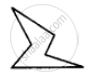is a closed curve entirely made up of line segments. The another name for this shape is ______.

Exercise | Q 63 | Page 151

A quadrilateral that is not a parallelogram but has exactly two opposite angles of equal measure is ______.

Exercise | Q 64 | Page 151

The measure of each angle of a regular pentagon is ______.

Exercise | Q 65 | Page 151

The name of three-sided regular polygon is ______.

Exercise | Q 66 | Page 151

The number of diagonals in a hexagon is ______.

Exercise | Q 67 | Page 151

A polygon is a simple closed curve made up of only ______.

Exercise | Q 68 | Page 151

A regular polygon is a polygon whose all sides are equal and all ______ are equal.

Exercise | Q 69 | Page 151

The sum of interior angles of a polygon of n sides is ______ right angles.

Exercise | Q 70 | Page 151

The sum of all exterior angles of a polygon is ______.

Exercise | Q 71 | Page 151

Exercise | Q 72 | Page 151

A quadrilateral in which a pair of opposite sides is parallel is ______.

Exercise | Q 73 | Page 151

If all sides of a quadrilateral are equal, it is a ______.

Exercise | Q 74 | Page 151

In a rhombus diagonals intersect at ______ angles.

Exercise | Q 75 | Page 151

______ measurements can determine a quadrilateral uniquely.

Exercise | Q 76 | Page 152

A quadrilateral can be constructed uniquely if its three sides and ______ angles are given.

Exercise | Q 77 | Page 152

A rhombus is a parallelogram in which ______ sides are equal.

Exercise | Q 78 | Page 152

The measure of ______ angle of concave quadrilateral is more than 180°.

Exercise | Q 79 | Page 152

A diagonal of a quadrilateral is a line segment that joins two ______ vertices of the quadrilateral.

Exercise | Q 80 | Page 152

The number of sides in a regular polygon having measure of an exterior angle as 72° is ______.

Exercise | Q 81 | Page 152

If the diagonals of a quadrilateral bisect each other, it is a ______.

Exercise | Q 82 | Page 152

The adjacent sides of a parallelogram are 5 cm and 9 cm. Its perimeter is ______.

Exercise | Q 83 | Page 152

A nonagon has ______ sides.

Exercise | Q 84 | Page 152

Diagonals of a rectangle are ______.

Exercise | Q 85 | Page 152

A polygon having 10 sides is known as ______.

Exercise | Q 86 | Page 152

A rectangle whose adjacent sides are equal becomes a ______.

Exercise | Q 87 | Page 152

If one diagonal of a rectangle is 6 cm long, length of the other diagonal is ______.

Exercise | Q 88 | Page 152

Adjacent angles of a parallelogram are ______.

Exercise | Q 89 | Page 152

If only one diagonal of a quadrilateral bisects the other, then the quadrilateral is known as ______.

Exercise | Q 90 | Page 152

In trapezium ABCD with AB || CD, if ∠A = 100°, then ∠D = ______.

Exercise | Q 91 | Page 152

The polygon in which sum of all exterior angles is equal to the sum of interior angles is called ______.

#### State whether the following statement is True or False:

Exercise | Q 92 | Page 152

All angles of a trapezium are equal.

• True

• False

Exercise | Q 93 | Page 152

All squares are rectangles.

• True

• False

Exercise | Q 94 | Page 152

All kites are squares.

• True

• False

Exercise | Q 95 | Page 152

All rectangles are parallelograms.

• True

• False

Exercise | Q 96 | Page 153

All rhombuses are squares.

• True

• False

Exercise | Q 97 | Page 153

Sum of all the angles of a quadrilateral is 180°.

• True

• False

Exercise | Q 98 | Page 153

• True

• False

Exercise | Q 99 | Page 153

Triangle is a polygon whose sum of exterior angles is double the sum of interior angles.

• True

• False

Exercise | Q 100 | Page 153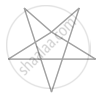is a polygon.

• True

• False

Exercise | Q 101 | Page 153

A kite is not a convex quadrilateral.

• True

• False

Exercise | Q 102 | Page 153

The sum of interior angles and the sum of exterior angles taken in an order are equal in case of quadrilaterals only.

• True

• False

Exercise | Q 103 | Page 153

If the sum of interior angles is double the sum of exterior angles taken in an order of a polygon, then it is a hexagon.

• True

• False

Exercise | Q 104 | Page 153

A polygon is regular if all of its sides are equal.

• True

• False

Exercise | Q 105 | Page 153

• True

• False

Exercise | Q 106 | Page 153

If diagonals of a quadrilateral are equal, it must be a rectangle.

• True

• False

Exercise | Q 107 | Page 153

If opposite angles of a quadrilateral are equal, it must be a parallelogram.

• True

• False

Exercise | Q 108 | Page 153

The interior angles of a triangle are in the ratio 1:2:3, then the ratio of its exterior angles is 3:2:1.

• True

• False

Exercise | Q 109 | Page 153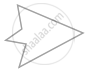is a concave pentagon

• True

• False

Exercise | Q 110 | Page 153

Diagonals of a rhombus are equal and perpendicular to each other.

• True

• False

Exercise | Q 111 | Page 153

Diagonals of a rectangle are equal.

• True

• False

Exercise | Q 112 | Page 153

Diagonals of rectangle bisect each other at right angles.

• True

• False

Exercise | Q 113 | Page 153

Every kite is a parallelogram.

• True

• False

Exercise | Q 114 | Page 154

Every trapezium is a parallelogram.

• True

• False

Exercise | Q 115 | Page 154

Every parallelogram is a rectangle.

• True

• False

Exercise | Q 116 | Page 154

Every trapezium is a rectangle.

• True

• False

Exercise | Q 117 | Page 154

Every rectangle is a trapezium.

• True

• False

Exercise | Q 118 | Page 154

Every square is a rhombus.

• True

• False

Exercise | Q 119 | Page 154

Every square is a parallelogram.

• True

• False

Exercise | Q 120 | Page 154

Every square is a trapezium.

• True

• False

Exercise | Q 121 | Page 154

Every rhombus is a trapezium

• True

• False

Exercise | Q 122 | Page 154

A quadrilateral can be drawn if only measures of four sides are given.

• True

• False

Exercise | Q 123 | Page 154

A quadrilateral can have all four angles as obtuse.

• True

• False

Exercise | Q 124 | Page 154

A quadrilateral can be drawn if all four sides and one diagonal is known.

• True

• False

Exercise | Q 125 | Page 154

A quadrilateral can be drawn when all the four angles and one side is given.

• True

• False

Exercise | Q 126 | Page 154

A quadrilateral can be drawn if all four sides and one angle is known.

• True

• False

Exercise | Q 127 | Page 154

A quadrilateral can be drawn if three sides and two diagonals are given.

• True

• False

Exercise | Q 128 | Page 154

If diagonals of a quadrilateral bisect each other, it must be a parallelogram.

• True

• False

Exercise | Q 129 | Page 154

A quadrilateral can be constructed uniquely if three angles and any two sides are given.

• True

• False

Exercise | Q 130 | Page 154

A parallelogram can be constructed uniquely if both diagonals and the angle between them is given.

• True

• False

Exercise | Q 131 | Page 154

A rhombus can be constructed uniquely if both diagonals are given.

• True

• False

#### Solve the following:

Exercise | Q 132 | Page 154

The diagonals of a rhombus are 8 cm and 15 cm. Find its side.

Exercise | Q 133 | Page 154

Two adjacent angles of a parallelogram are in the ratio 1:3. Find its angles.

Exercise | Q 134 | Page 154

Of the four quadrilaterals-square, rectangle, rhombus and trapezium-one is somewhat different from the others because of its design. Find it and give justification.

Exercise | Q 135 | Page 155

In a rectangle ABCD, AB = 25 cm and BC = 15. In what ratio does the bisector of ∠C divide AB?

Exercise | Q 136 | Page 155

PQRS is a rectangle. The perpendicular ST from S on PR divides ∠S in the ratio 2:3. Find ∠TPQ.

Exercise | Q 137 | Page 155

A photo frame is in the shape of a quadrilateral. With one diagonal longer than the other. Is it a rectangle? Why or why not?

Exercise | Q 138 | Page 155

The adjacent angles of a parallelogram are (2x – 4)° and (3x – 1)°. Find the measures of all angles of the parallelogram.

Exercise | Q 139 | Page 155

The point of intersection of diagonals of a quadrilateral divides one diagonal in the ratio 1:2. Can it be a parallelogram? Why or why not?

Exercise | Q 140 | Page 155

The ratio between exterior angle and interior angle of a regular polygon is 1:5. Find the number of sides of the polygon.

Exercise | Q 141 | Page 155

Two sticks each of length 5 cm are crossing each other such that they bisect each other. What shape is formed by joining their endpoints? Give reason.

Exercise | Q 142 | Page 155

Two sticks each of length 7 cm are crossing each other such that they bisect each other at right angles. What shape is formed by joining their end points? Give reason.

Exercise | Q 143 | Page 155

A playground in the town is in the form of a kite. The perimeter is 106 metres. If one of its sides is 23 metres, what are the lengths of other three sides?

Exercise | Q 144 | Page 155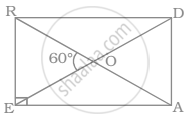Exercise | Q 145 | Page 156

In rectangle PAIR, find ∠ARI, ∠RMI and ∠PMA.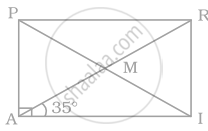Exercise | Q 146 | Page 156

In parallelogram ABCD, find ∠B, ∠C and ∠D.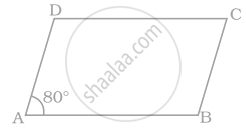Exercise | Q 147 | Page 156

In parallelogram PQRS, O is the mid point of SQ. Find ∠S, ∠R, PQ, QR and diagonal PR.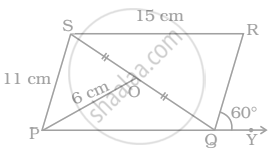Exercise | Q 148 | Page 156

In rhombus BEAM, find ∠AME and ∠AEM.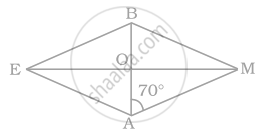Exercise | Q 149 | Page 156

In parallelogram FIST, find ∠SFT, ∠OST and ∠STO.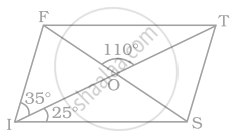Exercise | Q 150 | Page 157

In the given parallelogram YOUR, ∠RUO = 120° and OY is extended to point S such that ∠SRY = 50°. Find ∠YSR.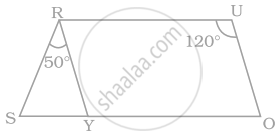Exercise | Q 151 | Page 157

In kite WEAR, ∠WEA = 70° and ∠ARW = 80°. Find the remaining two angles.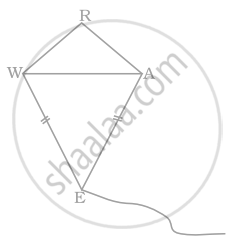Exercise | Q 152.(i) | Page 157

A rectangular MORE is shown below: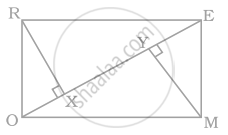Answer the following questions by giving appropriate reason.
Is RE = OM?

Exercise | Q 152.(ii) | Page 157

A rectangular MORE is shown below:Answer the following questions by giving appropriate reason.
Is ∠MYO = ∠RXE?

Exercise | Q 152.(iii) | Page 157

A rectangular MORE is shown below:Answer the following questions by giving appropriate reason.
Is ∠MOY = ∠REX?

Exercise | Q 152.(iv) | Page 157

A rectangular MORE is shown below:Answer the following questions by giving appropriate reason.
Is ∆MYO ≅ ∆RXE?

Exercise | Q 152.(v) | Page 157

A rectangular MORE is shown below:Answer the following questions by giving appropriate reason.
Is MY = RX?

Exercise | Q 153 | Page 158

In parallelogram LOST, SN⊥OL and SM⊥LT. Find ∠STM, ∠SON and ∠NSM.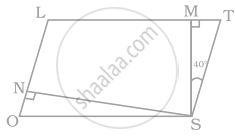Exercise | Q 154 | Page 158

In trapezium HARE, EP and RP are bisectors of ∠E and ∠R respectively. Find ∠HAR and ∠EHA.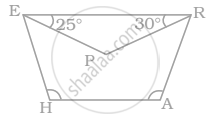Exercise | Q 155 | Page 158

In parallelogram MODE, the bisector of ∠M and ∠O meet at Q, find the measure of ∠MQO.

Exercise | Q 156 | Page 158

A playground is in the form of a rectangle ATEF. Two players are standing at the points F and B where EF = EB. Find the values of x and y.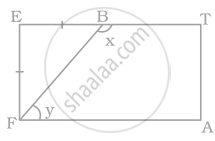Exercise | Q 157 | Page 158

In the following figure of a ship, ABDH and CEFG are two parallelograms. Find the value of x.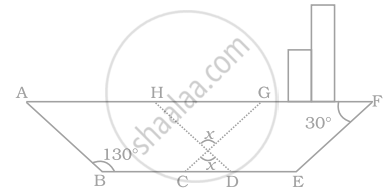Exercise | Q 158 | Page 159

A Rangoli has been drawn on a flor of a house. ABCD and PQRS both are in the shape of a rhombus. Find the radius of semicircle drawn on each side of rhombus ABCD.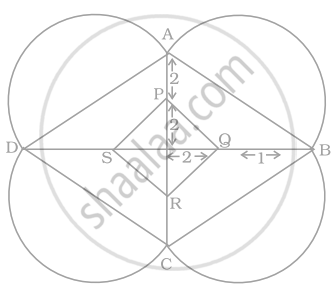Exercise | Q 159 | Page 159

ABCDE is a regular pentagon. The bisector of angle A meets the side CD at M. Find ∠AMC.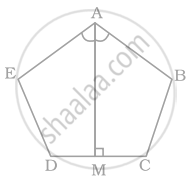Exercise | Q 160 | Page 159

Quadrilateral EFGH is a rectangle in which J is the point of intersection of the diagonals. Find the value of x if JF = 8x + 4 and EG = 24x – 8.

Exercise | Q 161 | Page 159

Find the values of x and y in the following parallelogram.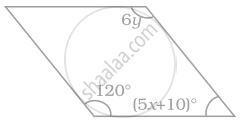Exercise | Q 162 | Page 160

Find the values of x and y in the following kite.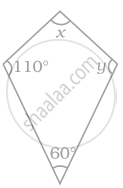Exercise | Q 163 | Page 160

Find the value of x in the trapezium ABCD given below.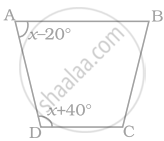Exercise | Q 164 | Page 160

Two angles of a quadrilateral are each of measure 75° and the other two angles are equal. What is the measure of these two angles? Name the possible figures so formed.

Exercise | Q 165 | Page 160

In a quadrilateral PQRS, ∠P = 50°, ∠Q = 50°, ∠R = 60°. Find ∠S. Is this quadrilateral convex or concave?

Exercise | Q 166 | Page 160

Both the pairs of opposite angles of a quadrilateral are equal and supplementary. Find the measure of each angle.

Exercise | Q 167 | Page 160

Find the measure of each angle of a regular octagon.

Exercise | Q 168 | Page 160

Find the measure of an are exterior angle of a regular pentagon and an exterior angle of a regular decagon. What is the ratio between these two angles?

Exercise | Q 169 | Page 160

In the figure, find the value of x.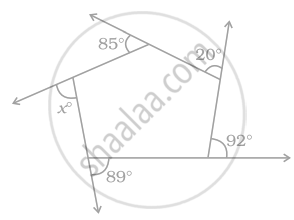Exercise | Q 170 | Page 161

Three angles of a quadrilateral are equal. Fourth angle is of measure 120°. What is the measure of equal angles?

Exercise | Q 171 | Page 161

In a quadrilateral HOPE, PS and ES are bisectors of ∠P and ∠E respectively. Give reason

Exercise | Q 172 | Page 161

ABCD is a parallelogram. Find the value of x, y and z.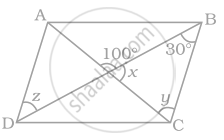Exercise | Q 173 | Page 161

Diagonals of a quadrilateral are perpendicular to each other. Is such a quadrilateral always a rhombus? Give a figure to justify your answer.

Exercise | Q 174 | Page 161

ABCD is a trapezium such that AB||CD, ∠A:∠D = 2 :1, ∠B:∠C = 7:5. Find the angles of the trapezium.

Exercise | Q 175 | Page 161

A line l is parallel to line m and a transversal p intersects them at X, Y respectively. Bisectors of interior angles at X and Y interesct at P and Q. Is PXQY a rectangle? Given reason.

Exercise | Q 176 | Page 161

ABCD is a parallelogram. The bisector of angle A intersects CD at X and bisector of angle C intersects AB at Y. Is AXCY a parallelogram? Give reason.

Exercise | Q 177 | Page 161

A diagonal of a parallelogram bisects an angle. Will it also bisect the other angle? Give reason.

Exercise | Q 178 | Page 161

The angle between the two altitudes of a parallelogram through the vertex of an obtuse angle of the parallelogram is 45°. Find the angles of the parallelogram.

Exercise | Q 179 | Page 161

ABCD is a rhombus such that the perpendicular bisector of AB passes through D. Find the angles of the rhombus.

Exercise | Q 180 | Page 161

ABCD is a parallelogram. Points P and Q are taken on the sides AB and AD respectively and the parallelogram PRQA is formed. If ∠C = 45°, find ∠R.

Exercise | Q 181 | Page 162

In parallelogram ABCD, the angle bisector of ∠A bisects BC. Will angle bisector of B also bisect AD? Give reason.

Exercise | Q 182 | Page 162

A regular pentagon ABCDE and a square ABFG are formed on opposite sides of AB. Find ∠BCF.

Exercise | Q 183 | Page 162

Find maximum number of acute angles which a convex, a quadrilateral, a pentagon and a hexagon can have. Observe the pattern and generalise the result for any polygon.

Exercise | Q 184 | Page 162

In the following figure, FD || BC || AE and AC || ED. Find the value of x.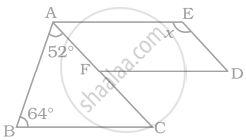Exercise | Q 185 | Page 162

In the following figure, AB || DC and AD = BC. Find the value of x.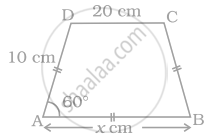Exercise | Q 186 | Page 162

Construct a trapezium ABCD in which AB||DC, ∠A = 105°, AD = 3 cm, AB = 4 cm and CD = 8 cm.

Exercise | Q 187 | Page 162

Construct a parallelogram ABCD in which AB = 4 cm, BC = 5 cm and ∠B = 60°.

Exercise | Q 188 | Page 162

Construct a rhombus whose side is 5 cm and one angle is of 60°.

Exercise | Q 189 | Page 162

Construct a rectangle whose one side is 3 cm and a diagonal equal to 5 cm

Exercise | Q 190 | Page 162

Construct a square of side 4 cm.

Exercise | Q 191 | Page 162

Construct a rhombus CLUE in which CL = 7.5 cm and LE = 6 cm.

Exercise | Q 192 | Page 162

Construct a quadrilateral BEAR in which BE = 6 cm, EA = 7 cm, RB = RE = 5 cm and BA = 9 cm. Measure its fourth side.

Exercise | Q 193 | Page 163

Construct a parallelogram POUR in which, PO = 5.5 cm, OU = 7.2 cm and ∠O = 70°.

Exercise | Q 194 | Page 163

Draw a circle of radius 3 cm and draw its diameter and label it as AC. Construct its perpendicular bisector and let it intersect the circle at B and D. What type of quadrilateral is ABCD? Justify your answer.

Exercise | Q 195 | Page 163

Construct a parallelogram HOME with HO = 6 cm, HE = 4 cm and OE = 3 cm.

Exercise | Q 196 | Page 163

Is it possible to construct a quadrilateral ABCD in which AB = 3 cm, BC = 4 cm, CD = 5.4 cm, DA = 5.9 cm and diagonal AC = 8 cm? If not, why?

Exercise | Q 197 | Page 163

Is it possible to construct a quadrilateral ROAM in which RO = 4 cm, OA = 5 cm, ∠O = 120°, ∠R = 105° and ∠A = 135°? If not, why?

Exercise | Q 198 | Page 163

Construct a square in which each diagonal is 5cm long.

Exercise | Q 199 | Page 163

Construct a quadrilateral NEWS in which NE = 7 cm, EW = 6 cm, ∠N = 60°, ∠E = 110° and ∠S = 85°.

Exercise | Q 200 | Page 163

Construct a parallelogram when one of its side is 4 cm and its two diagonals are 5.6 cm and 7 cm. Measure the other side.

Exercise | Q 201 | Page 163

Find the measure of each angle of a regular polygon of 20 sides?

Exercise | Q 202 | Page 163

Construct a trapezium RISK in which RI || KS, RI = 7 cm, IS = 5 cm, RK = 6.5 cm and ∠I = 60°.

Exercise | Q 203 | Page 163

Construct a trapezium ABCD where AB || CD, AD = BC = 3.2 cm, AB = 6.4 cm and CD = 9.6 cm. Measure ∠B and ∠A.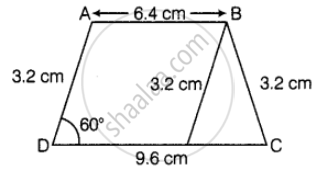## Chapter 5: Understanding Quadrilaterals and Practical Geometry

Exercise## NCERT solutions for Mathematics Exemplar Class 8 chapter 5 - Understanding Quadrilaterals and Practical Geometry

NCERT solutions for Mathematics Exemplar Class 8 chapter 5 (Understanding Quadrilaterals and Practical Geometry) include all questions with solution and detail explanation. This will clear students doubts about any question and improve application skills while preparing for board exams. The detailed, step-by-step solutions will help you understand the concepts better and clear your confusions, if any. Shaalaa.com has the CBSE Mathematics Exemplar Class 8 solutions in a manner that help students grasp basic concepts better and faster.

Further, we at Shaalaa.com provide such solutions so that students can prepare for written exams. NCERT textbook solutions can be a core help for self-study and acts as a perfect self-help guidance for students.